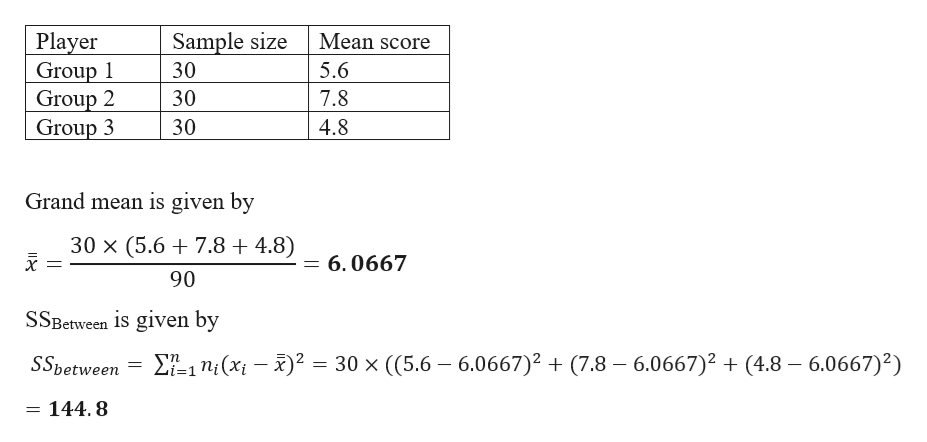# If the sample size is 30 and the mean score for group one is 5.6, mean score for group two Is 7.8, and mean score for group three is 4.8, what I the ss between of this study

Question
1 views

If the sample size is 30 and the mean score for group one is 5.6, mean score for group two Is 7.8, and mean score for group three is 4.8, what I the ss between of this study

check_circle

Step 1

Given data and calculati...help_outlineImage TranscriptionclosePlayer Group 1 Group 2 Group 3 Mean score Sample size 30 5.6 7.8 30 30 4.8 Grand mean is given by 30 x (5.6 7.8 + 4.8) 6.0667 90 SSBetween is given by ΣΕ n, (x3)2 30 x ((5.6 6.0667)2 (7.8 6.0667)2 (4.8 6.0667)2) SSbetween i=1 144.8 fullscreen

### Want to see the full answer?

See Solution

#### Want to see this answer and more?

Solutions are written by subject experts who are available 24/7. Questions are typically answered within 1 hour.*

See Solution
*Response times may vary by subject and question.
Tagged in

### Hypothesis Testing